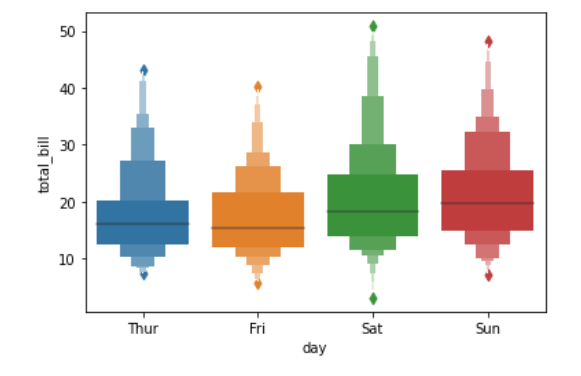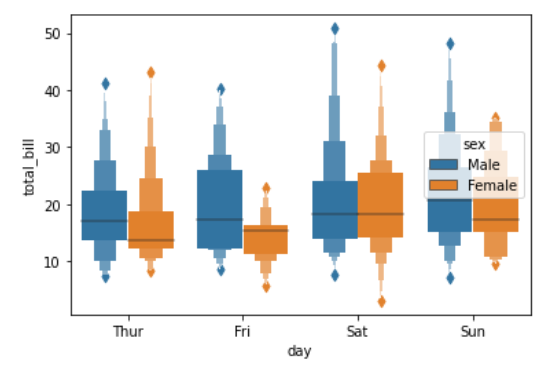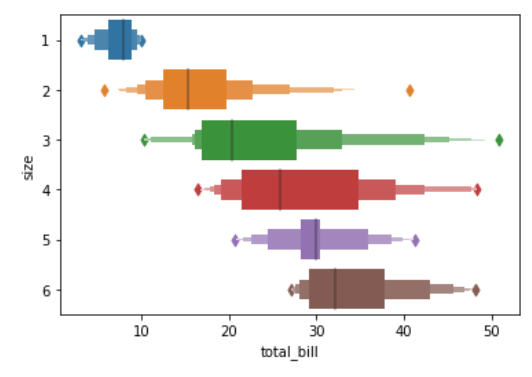Open in App
Not now

# Python – seaborn.boxenplot() method

• Last Updated : 18 Aug, 2020

Prerequisite : Fundamentals of Seaborn

Seaborn is a Python data visualization library based on matplotlib. It provides a high-level interface for drawing attractive and informative statistical graphics. There is just something extraordinary about a well-designed visualization. The colors stand out, the layers blend nicely together, the contours flow throughout, and the overall package not only has a nice aesthetic quality, but it provides meaningful insights to us as well.

## seaborn.boxenplot()

Draw an enhanced box plot for larger datasets. This style of plot was originally named a “letter value” plot because it shows a large number of quantiles that are defined as “letter values”.  It is similar to a box plot in plotting a nonparametric representation of a distribution in which all features correspond to actual observations. By plotting more quantiles, it provides more information about the shape of the distribution, particularly in the tails.

Syntax : seaborn.boxenplot(parameters)

Parameters :

• x, y, hue : Inputs for plotting long-form data.
• data : Dataset for plotting.
• order, hue_order : Order to plot the categorical levels in, otherwise the levels are inferred from the data objects.
• orient : Orientation of the plot (vertical or horizontal).
• color : Color for all of the elements, or seed for a gradient palette.
• palette : Colors to use for the different levels of the hue variable.
• saturation : Proportion of the original saturation to draw colors at.
• width : Width of a full element when not using hue nesting, or width of all the elements for one level of the major grouping variable.
• dodge : When hue nesting is used, whether elements should be shifted along the categorical axis.
• k_depth : The number of boxes, and by extension number of percentiles, to draw.
• linewidth : Width of the gray lines that frame the plot elements.
• scale : Method to use for the width of the letter value boxes.
• outlier_prop : Proportion of data believed to be outliers.
• showfliers : If False, suppress the plotting of outliers.
• ax : Axes object to draw the plot onto, otherwise uses the current Axes.
• kwargs : Other keyword arguments

Returns : Returns the Axes object with the plot drawn onto it.

Below is the implementation of above method with some examples :

Example 1:

 `# importing packages``import` `seaborn as sns``import` `matplotlib.pyplot as plt`` ` `# loading dataset``data ``=` `sns.load_dataset(``"tips"``)`` ` `# plot the boxenplot``sns.boxenplot(x ``=` `"day"``, y ``=` `"total_bill"``, ``              ``data ``=` `data)``plt.show()`

Output :Example 2:

 `# importing packages``import` `seaborn as sns``import` `matplotlib.pyplot as plt`` ` `# loading dataset``data ``=` `sns.load_dataset(``"tips"``)`` ` `# plot the boxenplot``# hue by sex``# width of 0.8``sns.boxenplot(x ``=``"day"``, y ``=` `"total_bill"``, hue ``=` `"sex"``, ``              ``data ``=` `data, width ``=` `0.8``)``plt.show()`

Output :Example 3:

 `# importing packages``import` `seaborn as sns``import` `matplotlib.pyplot as plt`` ` `# loading dataset``data ``=` `sns.load_dataset(``"tips"``)`` ` `# plot the boxenplot``# orient to horizontal``sns.boxenplot(x ``=` `"total_bill"``, y ``=` `"size"``, ``              ``data ``=` `data, orient ``=``"h"``)``plt.show()`

Output :My Personal Notes arrow_drop_up